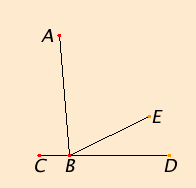# Proposition 14

If with any straight line, and at a point on it, two straight lines not lying on the same side make the sum of the adjacent angles equal to two right angles, then the two straight lines are in a straight line with one another.

With any straight line AB, and at the point B on it, let the two straight lines BC and BD not lying on the same side make the sum of the adjacent angles ABC and ABD equal to two right angles.

I say that BD is in a straight line with CB.I.Post.2

If BD is not in a straight line with BC, then produce BE in a straight line with CB.

Since the straight line AB stands on the straight line CBE, therefore the sum of the angles ABC and ABE equals two right angles. But the sum of the angles ABC and ABD also equals two right angles, therefore the sum of the angles CBA and ABE equals the sum of the angles CBA and ABD.

C.N.3

Subtract the angle CBA from each. Then the remaining angle ABE equals the remaining angle ABD, the less equals the greater, which is impossible. Therefore BE is not in a straight line with CB.

Similarly we can prove that neither is any other straight line except BD. Therefore CB is in a straight line with BD.

Therefore if with any straight line, and at a point on it, two straight lines not lying on the same side make the sum of the adjacent angles equal to two right angles, then the two straight lines are in a straight line with one another.

Q.E.D.

## Guide

This is a converse of the last proposition.

This is a proposition in plane geometry. If A, B, C, and D do not lie in a plane, then CBD cannot be a straight line. An ambient plane is necessary to talk about the sides of the line AB

The qualifying sentence, “Similarly we can prove that neither is any other straight line except BD,” is meant to take care of the cases when E does not lie inside the angle ABD.

This is the first use of Postulate 4 that all right angles are equal.

#### Use of Proposition 14

This proposition is used in propositions I.45, I.47, and a few in Books VI and XI.